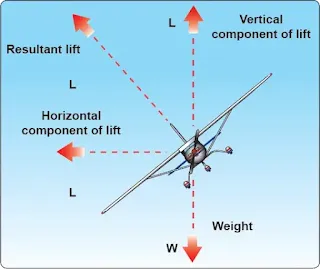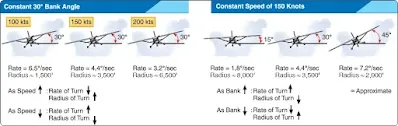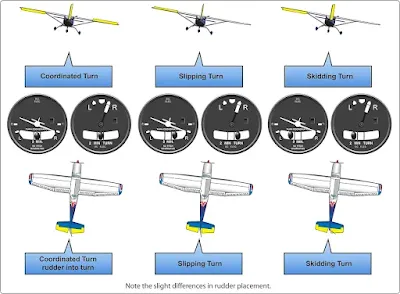# Climbs and Turns - Aerodynamic Factors

## Climbs

The ability for an aircraft to climb depends upon an excess power or thrust over what it takes to maintain equilibrium. Excess power is the available power over and above that required to maintain horizontal flight at a given speed. Although the terms power and thrust are sometimes used interchangeably (erroneously implying they are synonymous), distinguishing between the two is important when considering climb performance. Work is the product of a force moving through a distance and is usually independent of time. Power implies work rate or units of work per unit of time, and as such is a function of the speed at which the force is developed. Thrust, also a function of work, means the force which imparts a change in the velocity of a mass.

During takeoff, the aircraft does not stall even though it may be in a climb near the stall speed. The reason is that excess power (used to produce thrust) is used during this flight regime. Therefore, it is important if an engine fails after takeoff, to compensate the loss of thrust with pitch and airspeed.

For a given weight of the aircraft, the angle of climb depends on the difference between thrust and drag, or the excess thrust. When the excess thrust is zero, the inclination of the flightpath is zero, and the aircraft is in steady, level flight. When thrust is greater than drag, the excess thrust allows a climb angle depending on the amount of excess thrust. When thrust is less than drag, the deficiency of thrust induces an angle of descent.

### Acceleration in Cruise Flight

Aircraft accelerate in level flight because of an excess of power over what is required to maintain a steady speed. This is the same excess power used to climb. Upon reaching the desired altitude with pitch being lowered to maintain that altitude, the excess power now accelerates the aircraft to its cruise speed. However, reducing power too soon after level off results in a longer period of time to accelerate.

## Turns

Like any moving object, an aircraft requires a sideward force to make it turn. In a normal turn, this force is supplied by banking the aircraft in order to exert lift inward, as well as upward. The force of lift is separated into two components at right angles to each other. [Figure 1] The upward acting lift together with the opposing weight becomes the vertical lift component. The horizontally acting lift and its opposing centrifugal force are the horizontal lift component, or centripetal force. This horizontal lift component is the sideward force that causes an aircraft to turn. The equal and opposite reaction to this sideward force is centrifugal force, which is merely an apparent force as a result of inertia.Figure 1. Forces in a turn

The relationship between the aircraft’s speed and bank angle to the rate and radius of turns is important for instrument pilots to understand. The pilot can use this knowledge to properly estimate bank angles needed for certain rates of turn, or to determine how much to lead when intercepting a course.

### Rate of Turn

The rate of turn, normally measured in degrees per second, is based upon a set bank angle at a set speed. If either one of these elements changes, the rate of turn changes. If the aircraft increases its speed without changing the bank angle, the rate of turn decreases. Likewise, if the speed decreases without changing the bank angle, the rate of turn increases.

Changing the bank angle without changing speed also causes the rate of turn to change. Increasing the bank angle without changing speed increases the rate of turn, while decreasing the bank angle reduces the rate of turn.

The standard rate of turn, 3° per second, is used as the main reference for bank angle. Therefore, the pilot must understand how the angle of bank varies with speed changes, such as slowing down for holding or an instrument approach. Figure 2 shows the turn relationship with reference to a constant bank angle or a constant airspeed, and the effects on rate of turn and radius of turn. A rule of thumb for determining the standard rate turn is to divide the airspeed by ten and add 7. An aircraft with an airspeed of 90 knots takes a bank angle of 16° to maintain a standard rate turn (90 divided by 10 plus 7 equals 16°).Figure 2. Turns

The radius of turn varies with changes in either speed or bank. If the speed is increased without changing the bank angle, the radius of turn increases, and vice versa. If the speed is constant, increasing the bank angle reduces the radius of turn, while decreasing the bank angle increases the radius of turn. This means that intercepting a course at a higher speed requires more distance, and therefore, requires a longer lead. If the speed is slowed considerably in preparation for holding or an approach, a shorter lead is needed than that required for cruise flight.

### Coordination of Rudder and Aileron Controls

Any time ailerons are used, adverse yaw is produced. Adverse yaw is caused when the ailerons are deflected as a roll motion (as in turn) is initiated. In a right turn, the right aileron is deflected upward while the left is deflected downward. Lift is increased on the left side and reduced on the right, resulting in a bank to the right. However, as a result of producing lift on the left, induced drag is also increased on the left side. The drag causes the left wing to slow down, in turn causing the nose of the aircraft to initially move (left) in the direction opposite of the turn. Correcting for this yaw with rudder, when entering and exiting turns, is necessary for precise control of the airplane when flying on instruments. The pilot can tell if the turn is coordinated by checking the ball in the turn-and-slip indicator or the turn coordinator. [Figure 3]Figure 3. Adverse yaw

As the aircraft banks to enter a turn, a portion of the wing’s vertical lift becomes the horizontal component; therefore, without an increase in back pressure, the aircraft loses altitude during the turn. The loss of vertical lift can be offset by increasing the pitch in one-half bar width increments. Trim may be used to relieve the control pressures; however, if used, it has to be removed once the turn is complete.

In a slipping turn, the aircraft is not turning at the rate appropriate to the bank being used, and the aircraft falls to the inside of the turn. The aircraft is banked too much for the rate of turn, so the horizontal lift component is greater than the centrifugal force. A skidding turn results from excess of centrifugal force over the horizontal lift component, pulling the aircraft toward the outside of the turn. The rate of turn is too great for the angle of bank, so the horizontal lift component is less than the centrifugal force.

An inclinometer, located in the turn coordinator, or turn and bank indicator indicates the quality of the turn, and should be centered when the wings are banked. If the ball is off of center on the side toward the turn, the aircraft is slipping and rudder pressure should be added on that side to increase the rate of turn or the bank angle should be reduced. If the ball is off of center on the side away from the turn, the aircraft is skidding and rudder pressure toward the turn should be relaxed or the bank angle should be increased. If the aircraft is properly rigged, the ball should be in the center when the wings are level; use rudder and/or aileron trim if available.

The increase in induced drag (caused by the increase in AOA necessary to maintain altitude) results in a minor loss of airspeed if the power setting is not changed.

RELATED POSTS